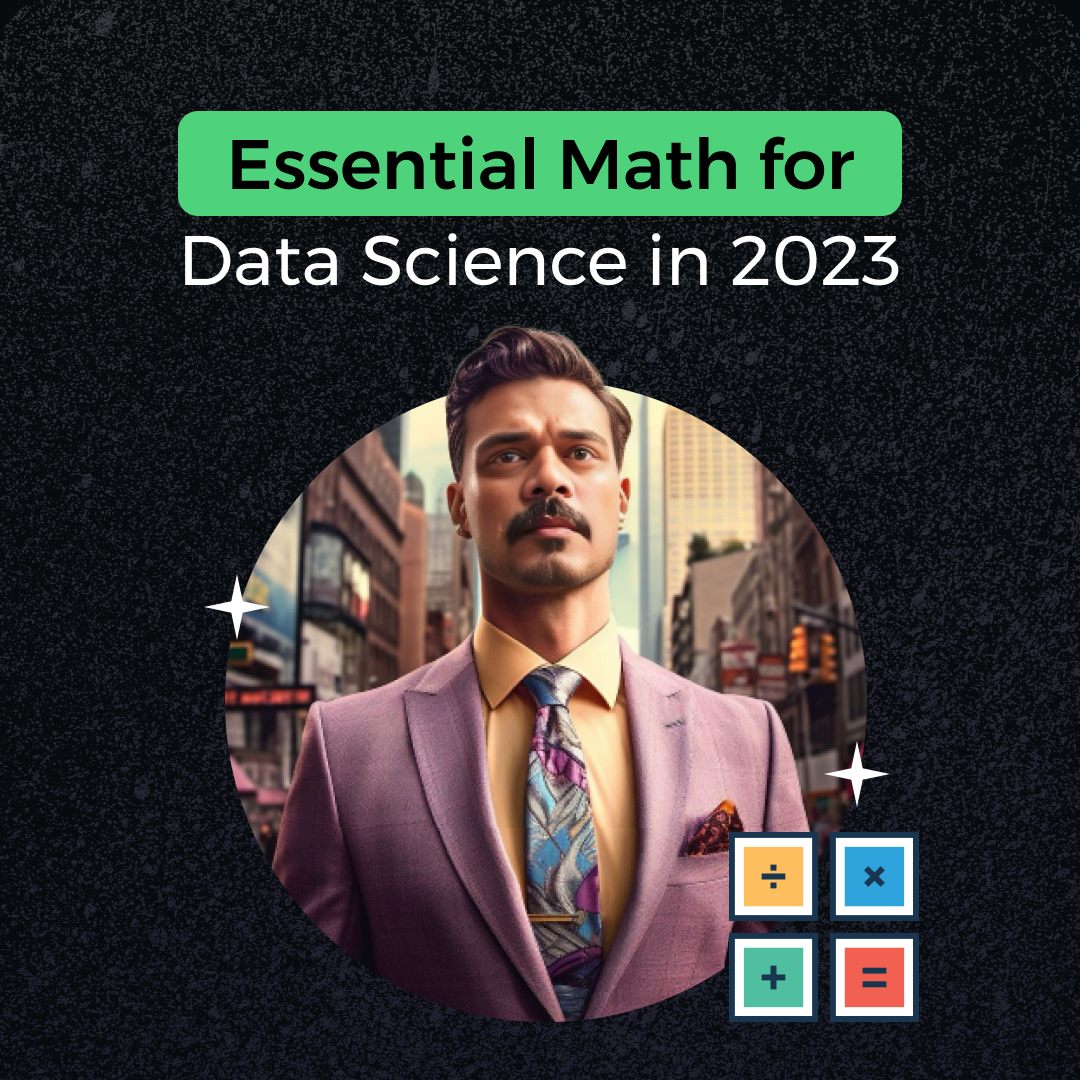# Essential Math for Data Science:A Beginner's GuideIn the realm of data science, mathematical concepts are the cornerstone that unlocks the vast potential hidden within datasets. However, for beginners, the prospect of delving into complex mathematical theories can appear daunting. In this comprehensive guide, we will explore the core mathematical pillars of data science: Linear Algebra, Statistics & Probability, and Calculus. We will also provide a curated list of resources to help you embark on your journey into the world of data science.

### Before We Begin: A Practical Approach to Learning

Before diving deep into the theoretical aspects of mathematics, especially if you are a beginner, it's important to emphasize a practical approach to learning. Instead of spending excessive time on theory, consider getting hands-on experience through course projects and real-world applications. This top-down approach encourages you to tackle problems first and then delve into the underlying mathematical concepts. This approach makes learning more meaningful and keeps you motivated to explore even further. Now, let's start with the first essential mathematical concept for data science: Linear Algebra.

### Linear Algebra

Imagine data as a vast, uncharted landscape, and Linear Algebra as the compass guiding you through it. In the physical world, distinguishing between a flower and a dog is straightforward, but teaching a computer to do the same is a complex task. Computers perceive images as collections of pixels, organized into matrices. Linear Algebra and matrices are essential tools for processing these images, identifying attributes like color, patterns, and shapes, and performing various operations on them.

Here are some excellent resources to start your journey in Linear Algebra:

Khan Academy Linear Algebra series (Beginner-friendly): Khan Academy offers a beginner-friendly introduction to Linear Algebra, perfect for those new to the field.

Course: Coding the Matrix: Linear Algebra Through Computer Science Application: This course bridges the gap between Linear Algebra and computer science applications, providing valuable insights into practical data manipulation.

YouTube: 3Blue1Brown Linear Algebra series: 3Blue1Brown's visually engaging videos make Linear Algebra concepts more intuitive and accessible.

Course: MIT Linear Algebra course: Explore Linear Algebra with the prestigious Massachusetts Institute of Technology through this comprehensive course.

Free E-book: Stanford CS229 Linear Algebra review: This resource is a great companion for reinforcing your understanding of Linear Algebra concepts.

### Statistics & Probability

Statistics and Probability are the twin stars illuminating the data science sky. Probability introduces the concept of uncertainty, a ubiquitous feature in real-world data. On the flip side, statistics transforms raw data into meaningful insights.

Here are some valuable resources to grasp Statistics & Probability:

Khan Academy Statistics and Probability series (Beginner-friendly): Khan Academy offers a beginner-friendly introduction to Statistics and Probability, making these concepts more approachable.

Seeing Theory: A visual introduction to probability and statistics: This resource provides a visual and intuitive introduction to probability and statistics, helping you build a strong foundation.

Course: Data Science Math skill by Duke University on Coursera: Duke University's course provides a comprehensive understanding of the mathematical skills crucial for data science.

Course: Intro to Descriptive Statistics &  Inferential Statistics from Udacity: Udacity's course is an excellent starting point to understand how to describe and summarize data.

Course: Statistics with R Specialization from Coursera: This specialization equips you with practical knowledge of statistics using the R programming language.

E-book: Stanford CS229 Probability Theory review: This E-book offers a comprehensive review of Probability Theory, enhancing your grasp of this essential concept.

### Calculus

Calculus is a powerful tool that helps us understand how things change and find optimal solutions in data science. It plays a crucial role in understanding data trends, training intelligent computer programs, and making informed decisions based on data.

Here are resources to start your journey in Calculus:

Khan Academy Calculus series (Beginner-friendly): Khan Academy's Calculus series provides a beginner-friendly introduction to Calculus concepts.

Youtube: 3Blue1Brown Calculus series: 3Blue1Brown's visually captivating videos make Calculus concepts more intuitive and accessible.

Coursera Mathematics for Machine Learning specialization: This Coursera specialization course introduces Calculus in the context of machine learning, bridging theory and practical application.

Course: MIT Single Variable Calculus: Explore Single Variable Calculus with the prestigious Massachusetts Institute of Technology.

Course: MIT Multivariable Calculus: This course covers Multivariable Calculus, an essential concept in data science.

Ebook: Stanford CS224n Differential Calculus review: This ebook provides a comprehensive review of Differential Calculus, enhancing your understanding of this fundamental mathematical tool.

Conclusion: Equipping Yourself for Data Science Success

In conclusion, understanding the fundamentals of Linear Algebra, Statistics & Probability, and Calculus equips you with the essential tools to navigate the complexities of data science. While the theoretical aspects are important, remember the value of practical, hands-on experience in real-world projects. This approach will make your learning more meaningful and motivate you to explore the depths of data science. We hope these resources cover all your needs and help you overcome any fear of mathematics on your journey to becoming a proficient data scientist. Happy learning!